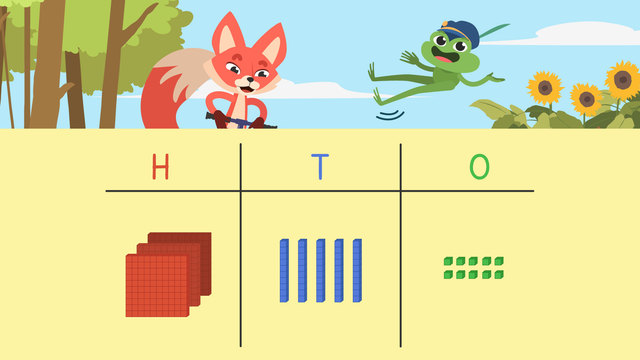# Place Value with 3 DigitsRate this video

Ø 5.0 / 1 ratings

The authorTeam Digital
Place Value with 3 Digits
CCSS.MATH.CONTENT.2.NBT.A.1

## Information about the videoPlace Value with 3 Digits

### In This Video on 3-Digit Place Value

Skylar and Henry are at a bingo hall with a twist - bingo is played by calling out the place value of 3-digit numbers! Skylar and Henry learn all about place value in 3-digit numbers so they can win the bingo prize.

### Place Value - 3-Digit Numbers Example

To read place value, we first look at each digit in a number. We can use a place value chart to help identify 3-digit number place value. Let’s look at the number two hundred and six, and use place value blocks to help.Place value blocks can help to understand place value! In the hundreds place, we write two hundreds because there is a two in the hundreds place. In the tens place we write zero tens because there is a zero in the tens place. Finally, in the ones place we write six ones because there is a six in the ones place.### Place Value with Three Digits - Review

Remember, in 3-digit numbers place value:

• The hundreds place shows how many hundreds there are.
• The tens place shows how many tens there are.
• The ones place shows how many ones there are.Below you will find some practice and a 3-digit place value worksheet.

### TranscriptPlace Value with 3 Digits

Skylar and Henry are at the bingo hall ready for place value bingo! The caller will shout out a number using place values, and Skylar and Henry will need to mark off the matching three digit number on their bingo cards! To help them play, let's learn about place value with three-digits. Place value is the value of a digit based on its position in a number. We can use a place value chart, and base ten blocks to help identify the value of a digit in a number. Let's look at the first number on Skylar's card: one hundred eleven. In the hundreds place value use one hundreds block, because the digit is one. We can also think of this as ten tens! The value of the hundreds place is one hundred In the tens place value use ones tens block because there is a one in the tens place. We can also think of this as ten ones! The value of the tens place is one ten. Finally, in the ones place value use one ones block because there is a one in the ones place. The value of the ones place is one one. Skylar will need to listen for one hundred plus one ten plus one one! Let's look at another number on Skylar's card! Here we have two hundred and six. In the hundreds place value use two hundreds blocks because there is a two in the hundreds place. What is the value of the hundreds place? The value is two hundreds. In the tens place value use zero tens blocks because the digit in the tens place is zero. What is the value of the tens place? The value is zero tens. Finally, in the ones place value use six ones blocks, because the digit in the ones place is six. What is the value of the ones place? The value is six ones. Skylar will need to listen out for two hundreds plus zero tens plus six ones! If the bingo caller says five hundreds plus six tens plus three ones, what three digit number will Skylar mark on her bingo card? There are five hundreds, six tens, and three ones. Skylar will mark five hundred sixty-three! While Skylar and Henry finish up playing place value bingo, let's review! Remember, base ten blocks can help identify the place value of numbers with three digits. The hundreds place shows how many hundreds there are. The tens place shows how many tens there are. The ones place shows how many ones there are. "I'm glad you won place value bingo, Skylar, that pogo stick is amazing!" "Me too because now, I'm just like you!"